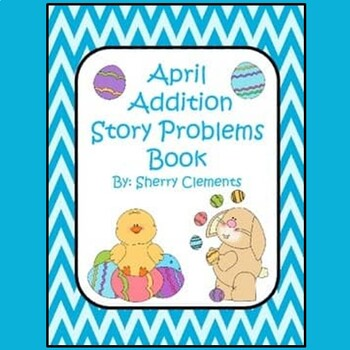# April Addition Story Problems Book (sums to 10)Grab this cute addition story problems book HERE!

This 25 page book is designed so that you can copy the book, cut it in half, staple, and then it is ready for use. Each page includes a number line 0-10, an addition story problem, and an equation matching the story problem. The 25 pages consist of a title page “My April Addition Book.” Then the remaining 24 pages actually include 12 different addition story problems. Each story problem is duplicated on the page following it. The first page for each story problem has the equation (addends) filled in with only the sum missing. The equation for the second page for each story problem only has the plus sign and equals sign in the equation with blank lines for students to fill in the missing addends from the story problem. The students must read the story problems and find the missing addends for the equation and then solve to find the sum. The number line is available to help students solve each problem. These two different page types allow for differentiation of students.
Some students are ready to read the word problem and find the sum while others rely on the equation being complete and only having to solve the equation.

These story problems can be put together in booklet form as discussed above or individual sheets can be used. The story problems are on a half sheet of paper.

These word problems are great for guided math groups, homework, math center activities, or morning work.

The preview shows each page in the packet.

I hope your students enjoy solving these story problems.

You may also like:
Subtraction Word Problems (Easter)
Subtraction Problems with Manipulatives (Creepy Crawlies)
Ten Frames 0-20 (April)
Morning Work - Kindergarten - April
Emergent Reader: Easter Eggs (number and color words)